# Area of a triangle Online Quiz

Following quiz provides Multiple Choice Questions (MCQs) related to Area of a triangle. You will have to read all the given answers and click over the correct answer. If you are not sure about the answer then you can check the answer using Show Answer button. You can use Next Quiz button to check new set of questions in the quiz.Q 1 - Find the area of the triangle given below.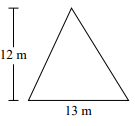### Explanation

Step 1:

Area of Right Triangle = $\frac{1}{2}$ × b × h; b = base = 13 m; h = height = 12 m

Step 2:

Area of Right Triangle = $\frac{1}{2}$ × 13 × 12 = 78 square m.

Q 2 - Find the area of the triangle given below.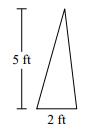### Explanation

Step 1:

Area of Right Triangle = $\frac{1}{2}$ × b × h; b = base = 2 ft m; h = height = 5 ft

Step 2:

Area of Right Triangle = $\frac{1}{2}$ × 2 × 5 = 5 square ft.

Q 3 - Find the area of the triangle given below.### Explanation

Step 1:

Area of Right Triangle = $\frac{1}{2}$ × b × h; b = base = 11 yd; h = height = 10 yd

Step 2:

Area of Right Triangle = $\frac{1}{2}$ × 11 × 10 = 55 square yd.

Q 4 - Find the area of the triangle given below.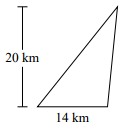### Explanation

Step 1:

Area of Right Triangle = $\frac{1}{2}$ × b × h; b = base = 14 km; h = height = 20 km

Step 2:

Area of Right Triangle = $\frac{1}{2}$ × 14 × 20 = 140 square km.

Q 5 - Find the area of the triangle given below.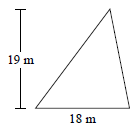### Explanation

Step 1:

Area of Right Triangle = $\frac{1}{2}$ × b × h; b = base = 18 m; h = height = 19 m

Step 2:

Area of Right Triangle = $\frac{1}{2}$ × 18 × 19 = 171 square m.

Q 6 - Find the area of the triangle given below.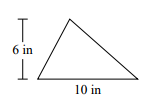### Explanation

Step 1:

Area of Right Triangle = $\frac{1}{2}$ × b × h; b = base = 10 in; h = height = 6 in

Step 2:

Area of Right Triangle = $\frac{1}{2}$ × 10 × 6 = 30 square in.

Q 7 - Find the area of the triangle given below.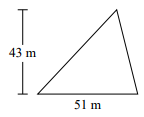### Explanation

Step 1:

Area of Right Triangle = $\frac{1}{2}$ × b × h; b = base = 51 m; h = height = 43 m

Step 2:

Area of Right Triangle = $\frac{1}{2}$ × 51 × 43 = 1096.5 square m.

Q 8 - Find the area of the triangle given below.### Explanation

Step 1:

Area of Right Triangle = $\frac{1}{2}$ × b × h; b = base = 3 yd; h = height = 6 yd

Step 2:

Area of Right Triangle = $\frac{1}{2}$ × 3 × 6 = 9 square yd.

Q 9 - Find the area of the triangle given below.### Explanation

Step 1:

Area of Right Triangle = $\frac{1}{2}$ × b × h; b = base = 4 ft; h = height = 8 ft

Step 2:

Area of Right Triangle = $\frac{1}{2}$ × 4 × 8 = 16 square ft.

Q 10 - Find the area of the triangle given below.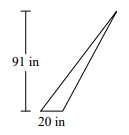### Explanation

Step 1:

Area of Right Triangle = $\frac{1}{2}$ × b × h; b = base = 20 in; h = height = 91 in

Step 2:

Area of Right Triangle = $\frac{1}{2}$ × 20 × 91 = 910 sq in.

area_of_triangle.htm